# ICSE Class 8 Maths Selina Solutions Chapter 11 Algebraic Expressions

The ICSE Class 8 Selina Solutions are very helpful for the students especially when they are preparing for the annual exams. Many times students are not able to solve the problems provided in the exercise. So, they need some authentic resources from where they can see the solutions and understand them. To help students with this we have provided the ICSE Class 8 Maths Selina Solutions.

Chapter 11 of ICSE Class 8 Maths covers the Algebraic Expressions. In this chapter, students will study how to find the coefficients and degree of a polynomial, addition and subtraction of polynomials and few word problems related to it. Here we have provided the step by step solution to all the questions in PDF format for ICSE Class 8 Maths Selina Solutions Chapter 11 Algebraic Expressions. Going through it students can easily understand the solution. To access the pdf click on the download link below;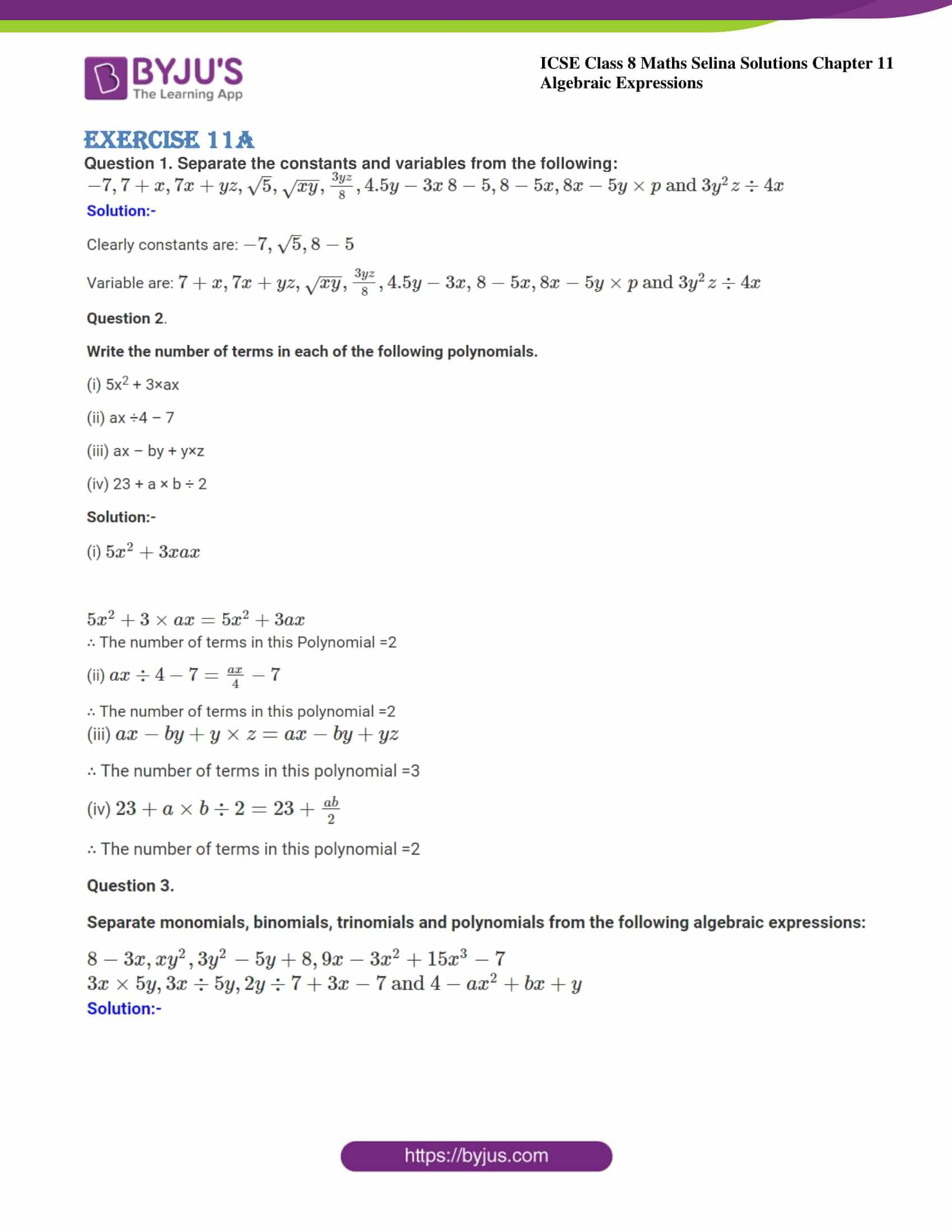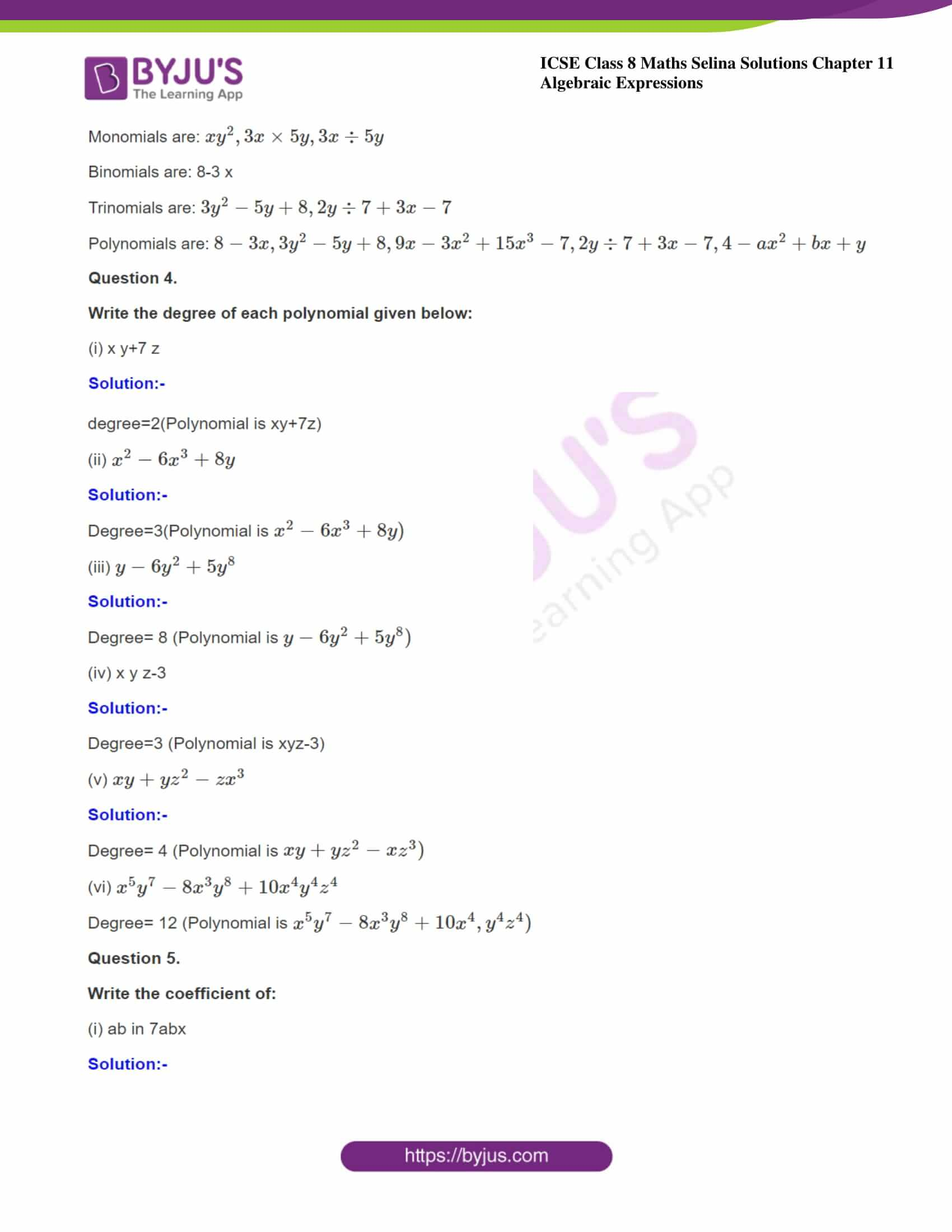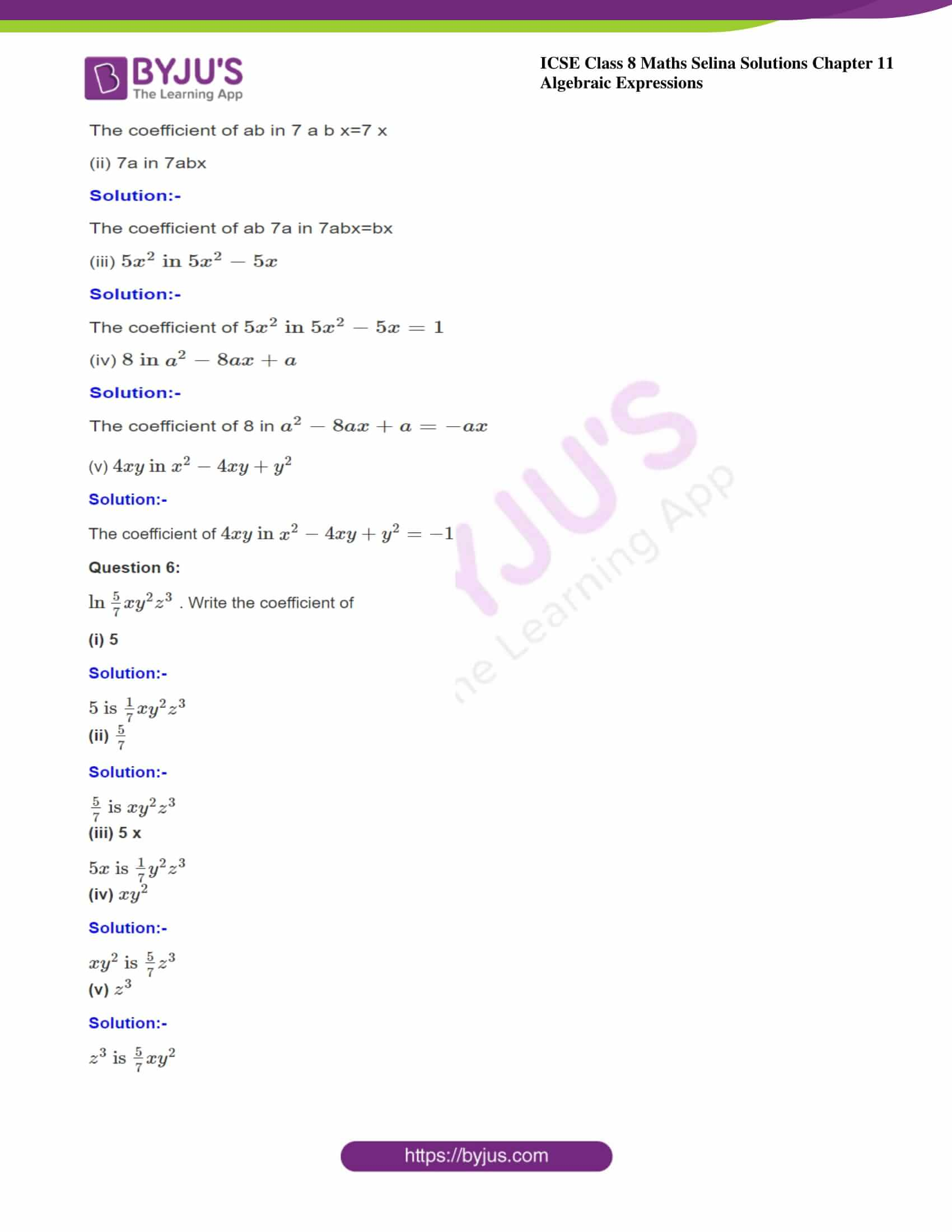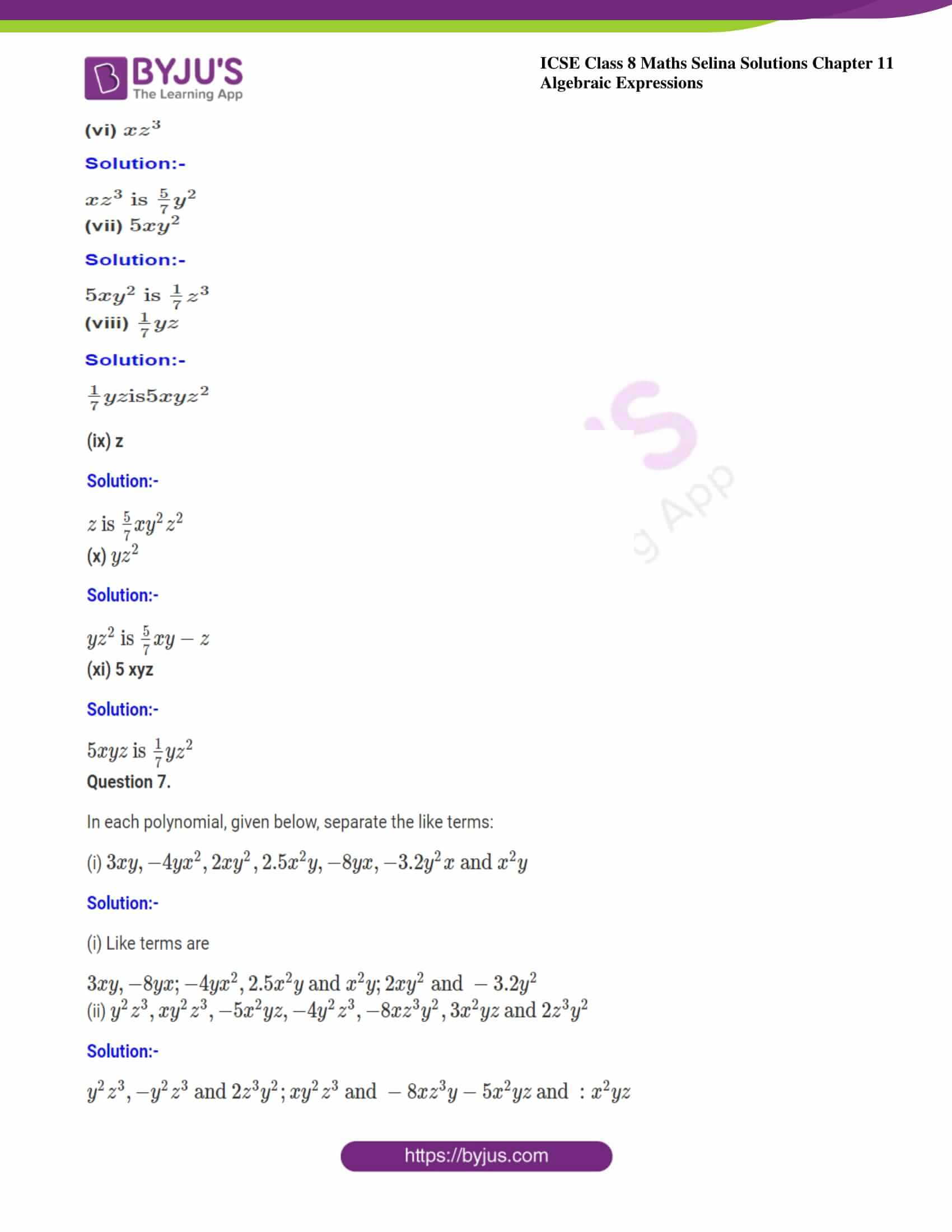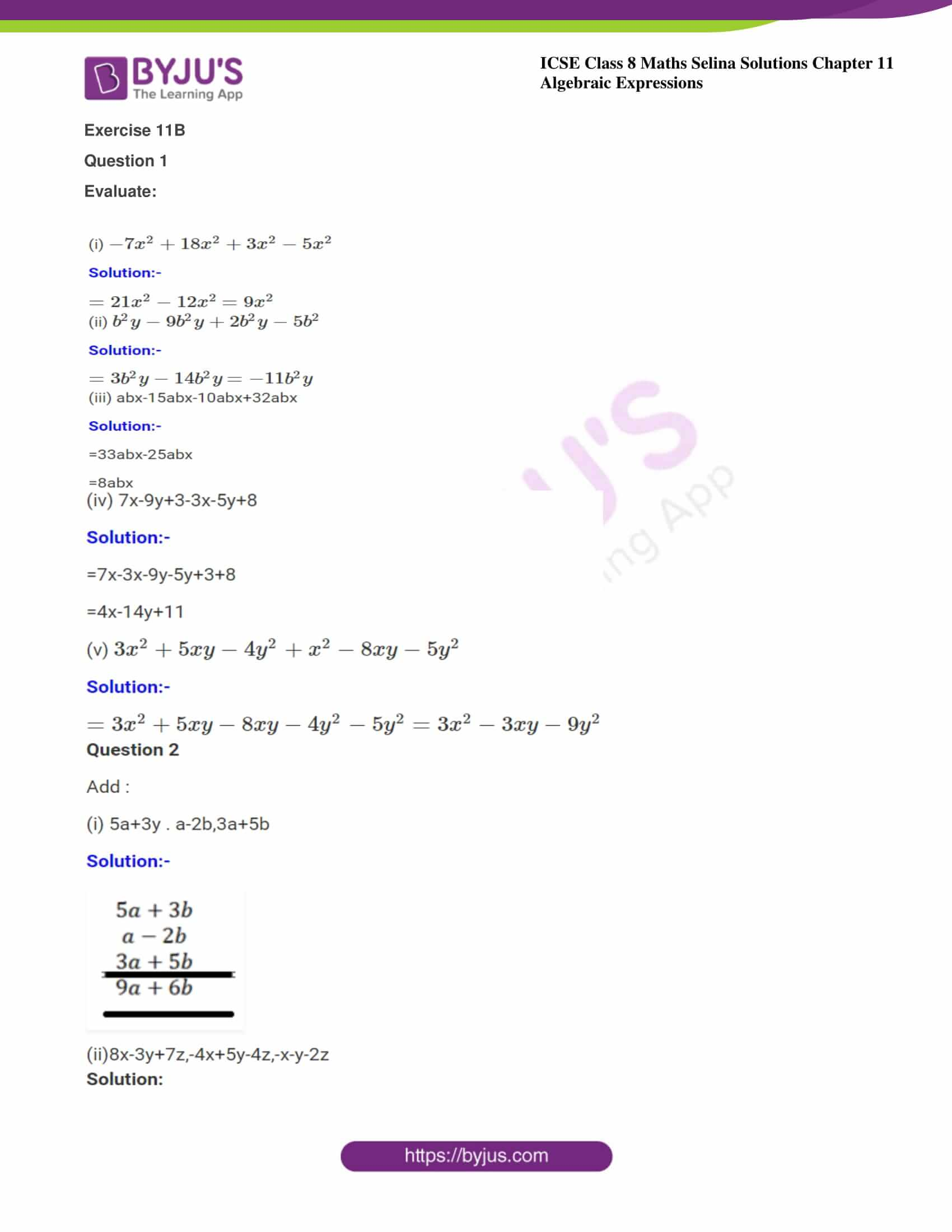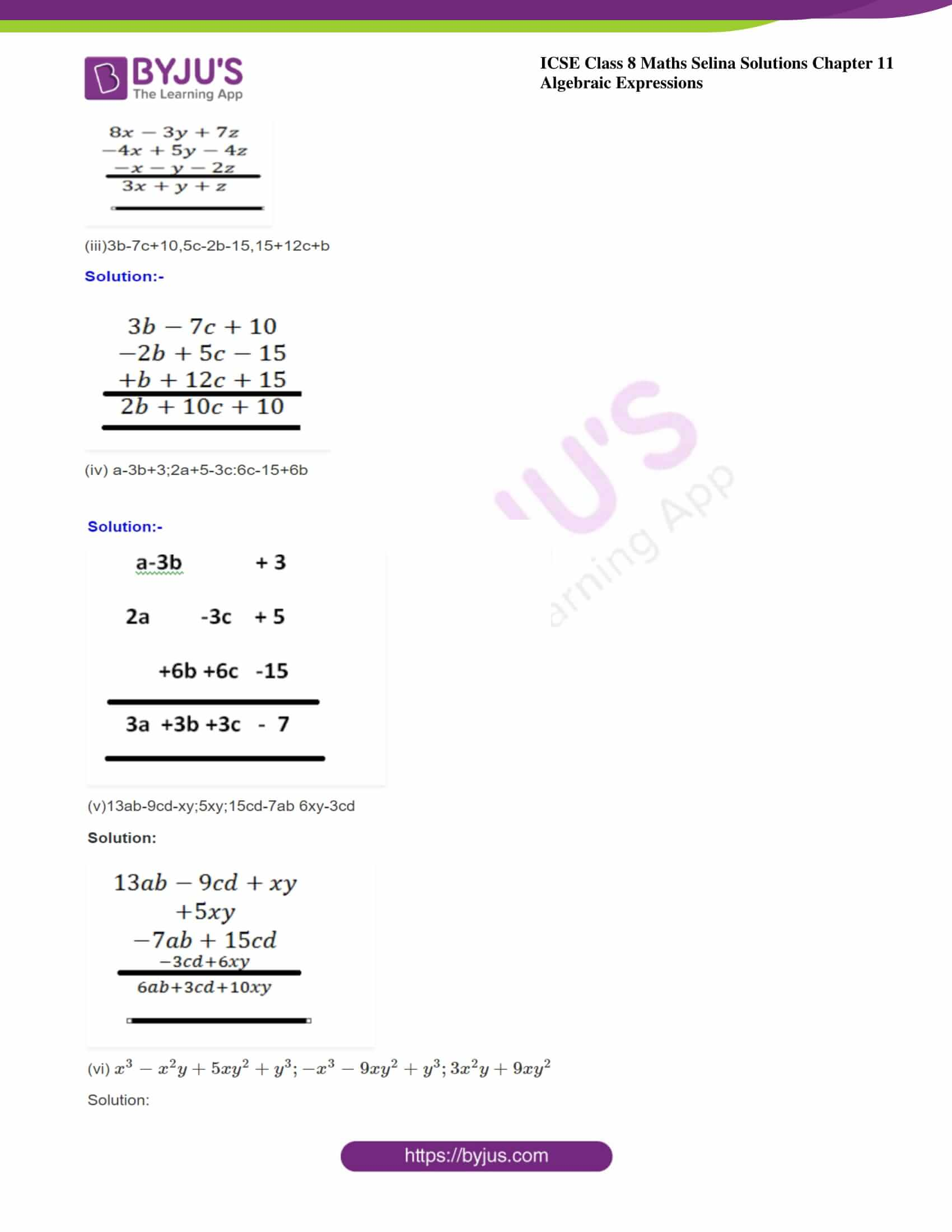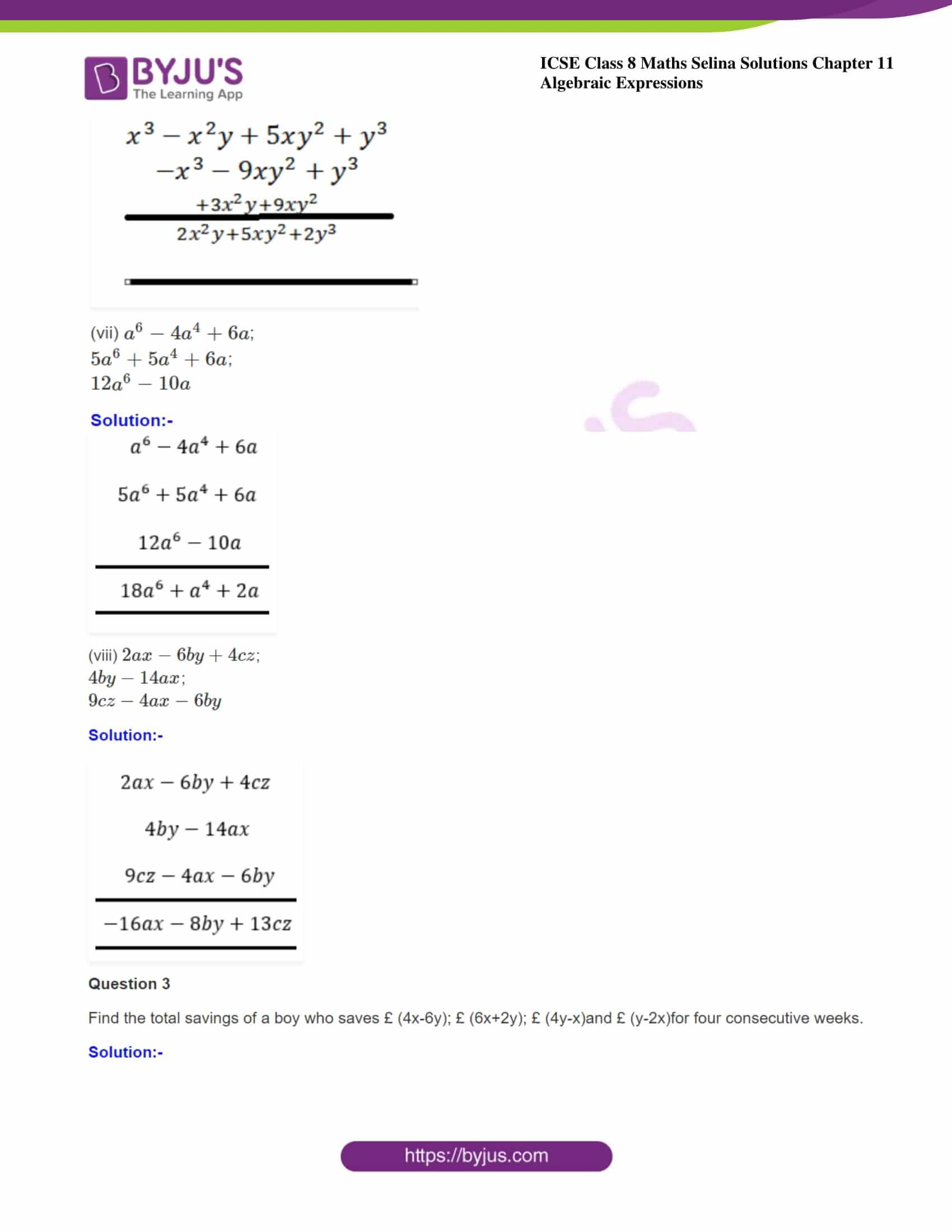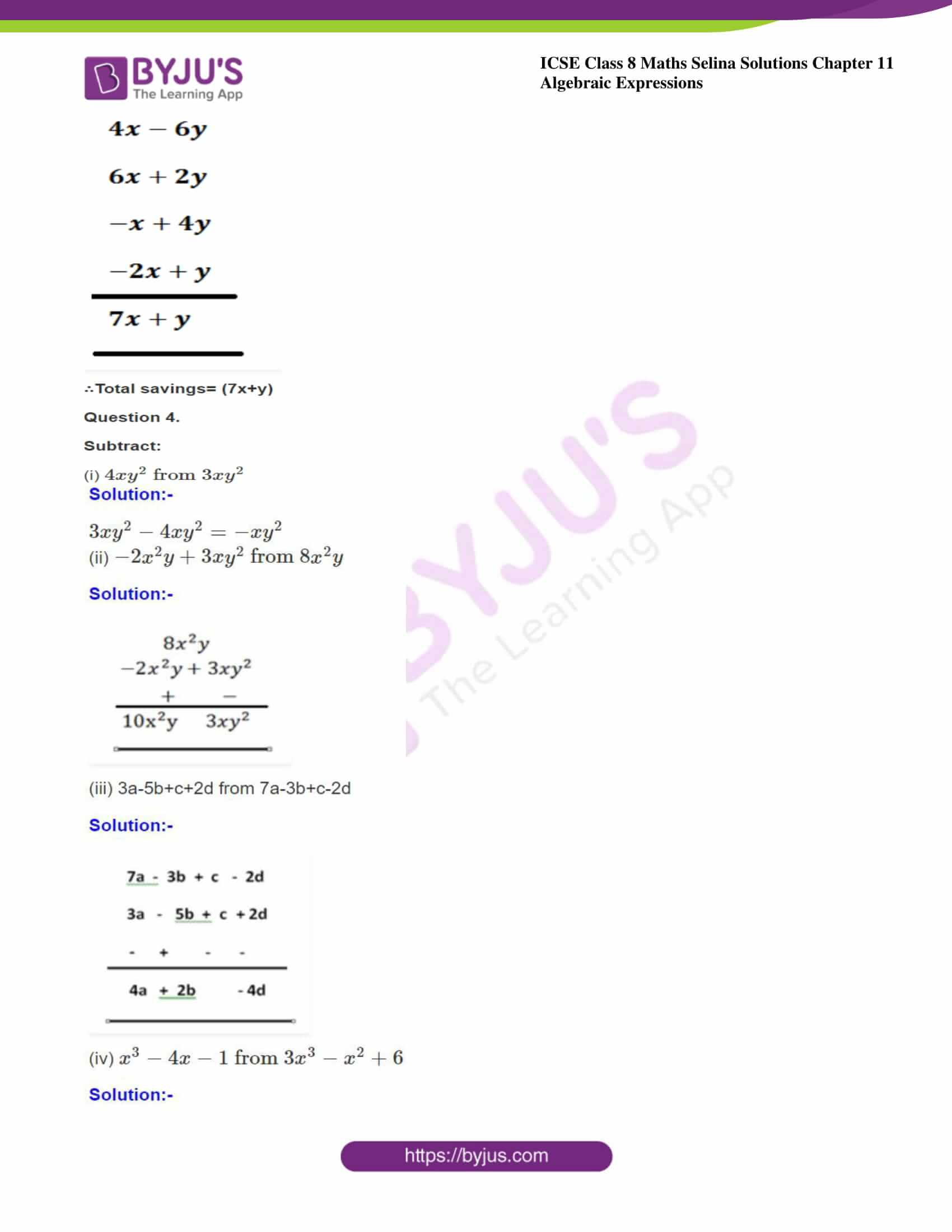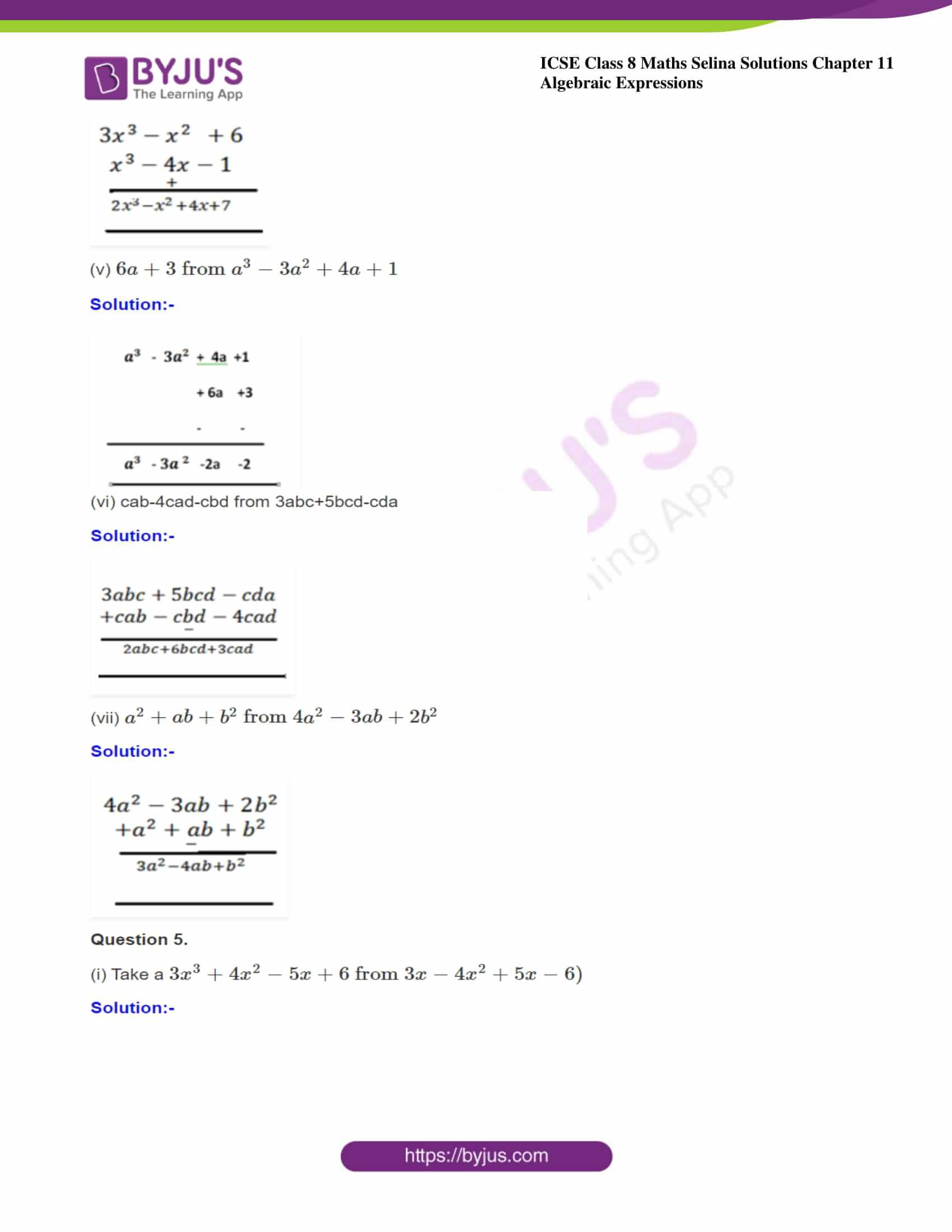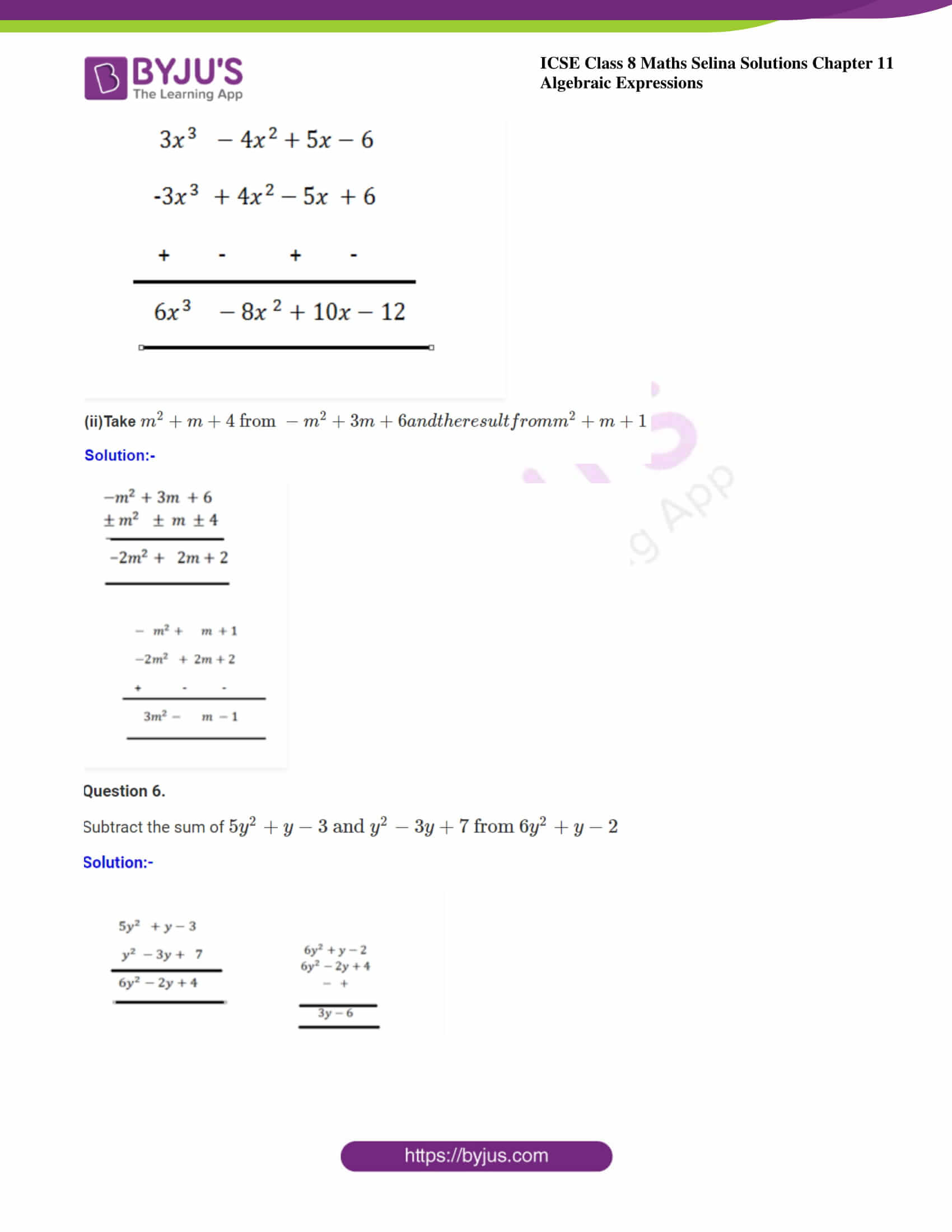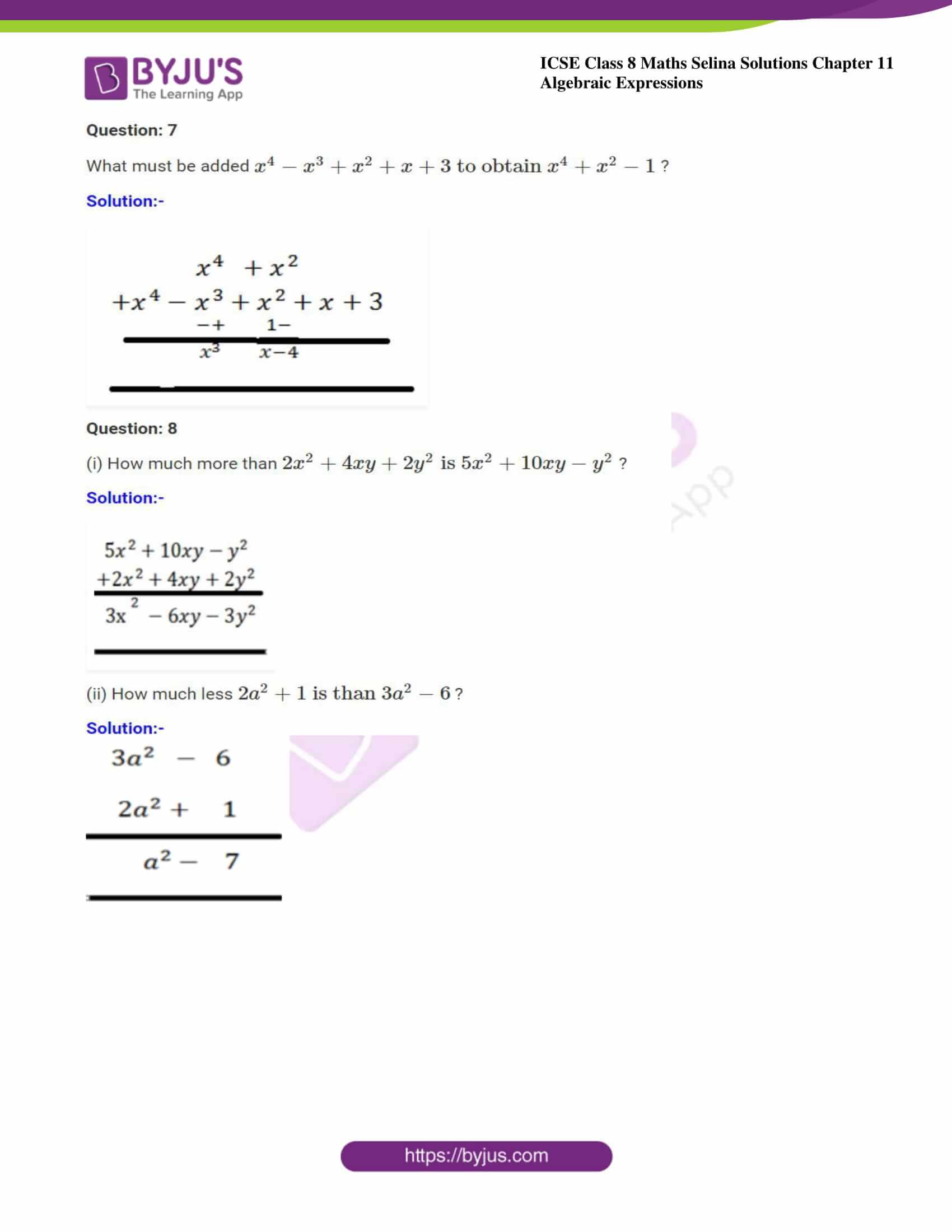ICSE Class 8 Maths Chapter 11 Algebraic Expressions has only 8 Questions. The solutions to all these questions are also provided below:

### ICSE Class 8 Maths Selina Solutions Chapter 11 – Algebraic Expressions

Exercise 11A

Question 1. Separate the constants and variables from the following: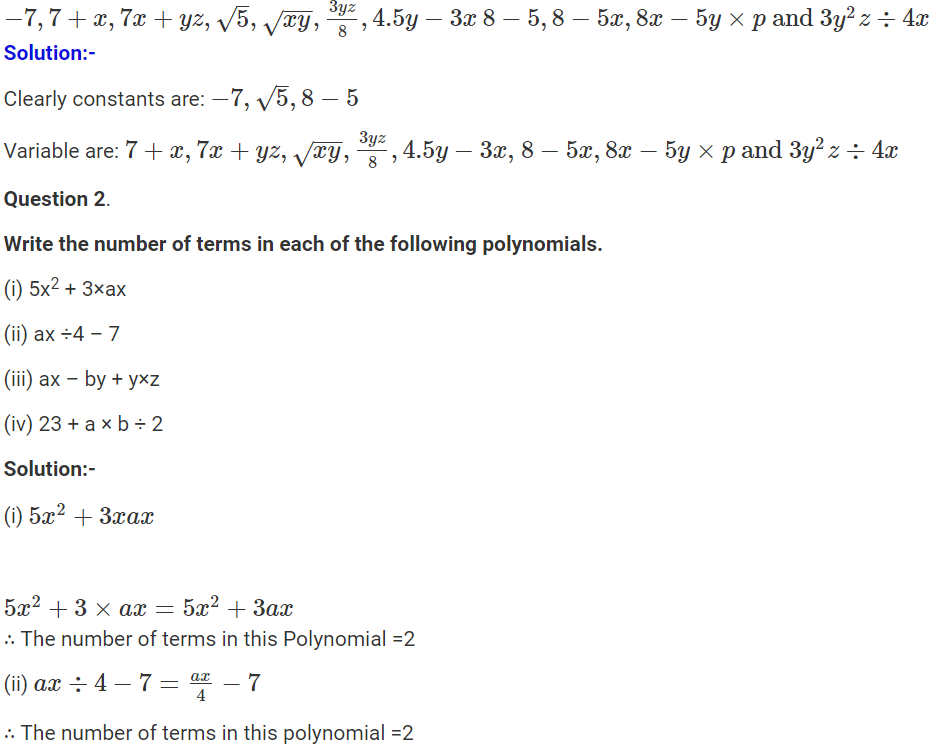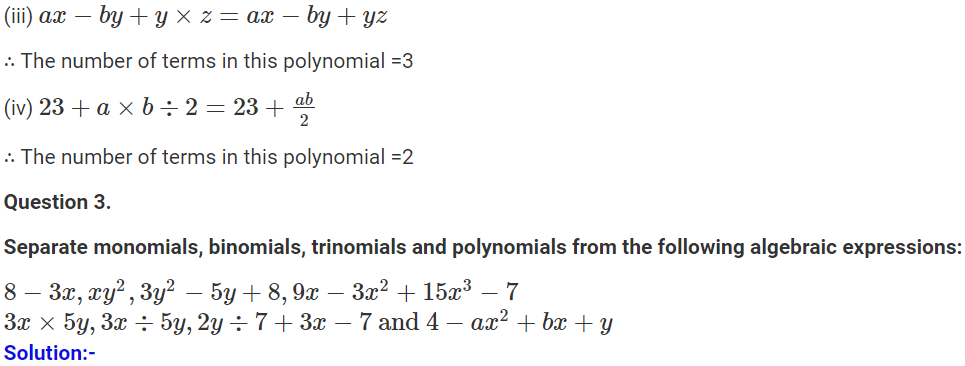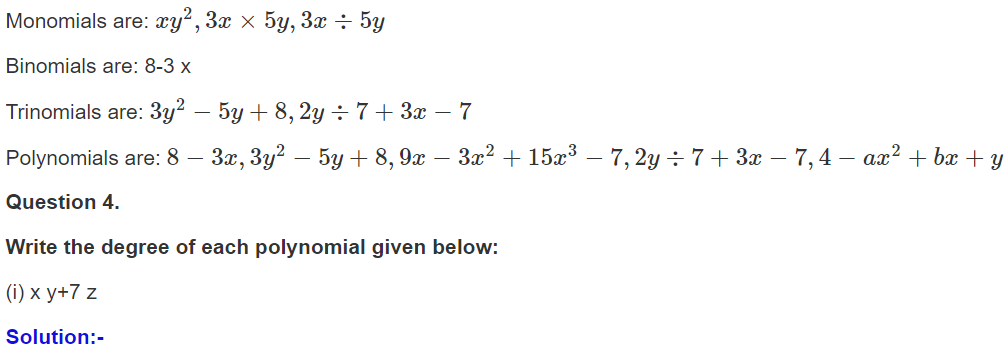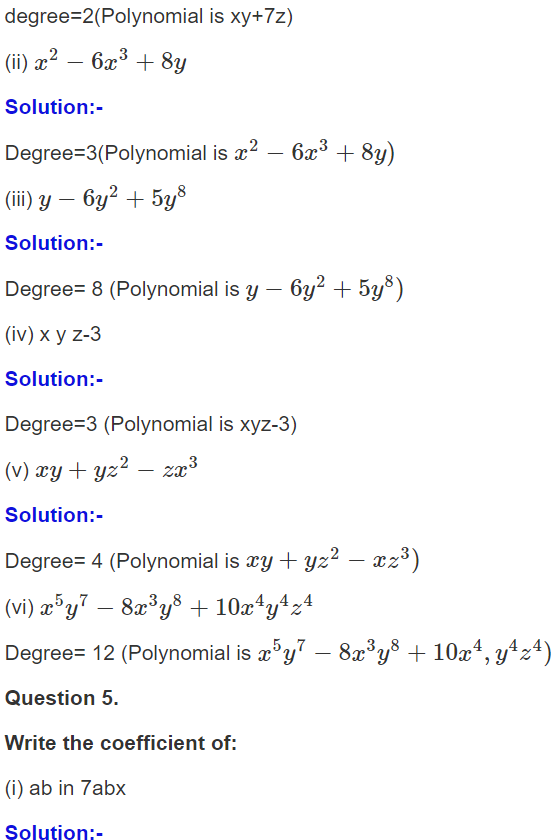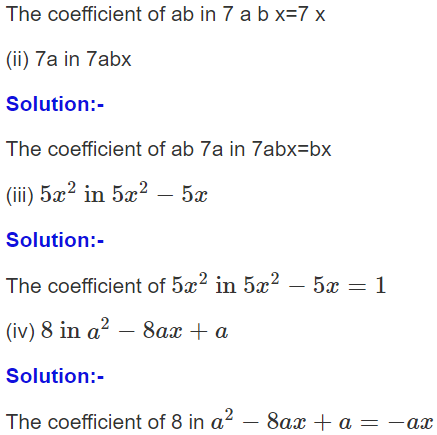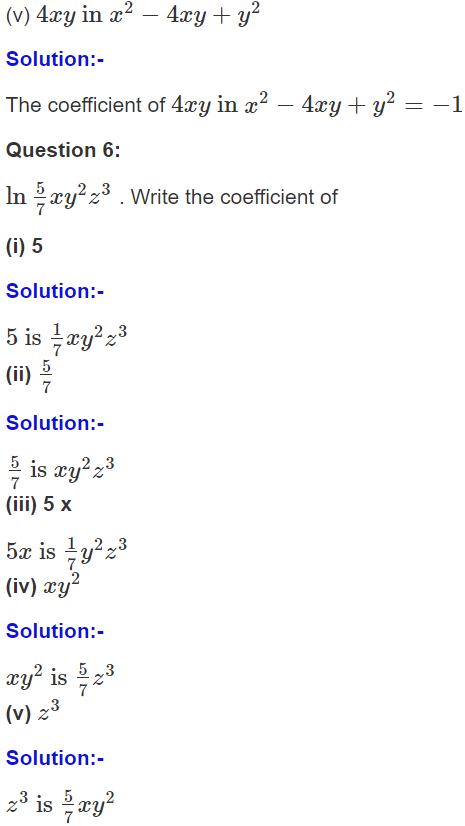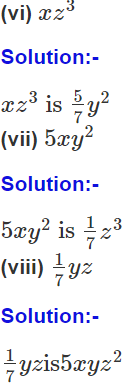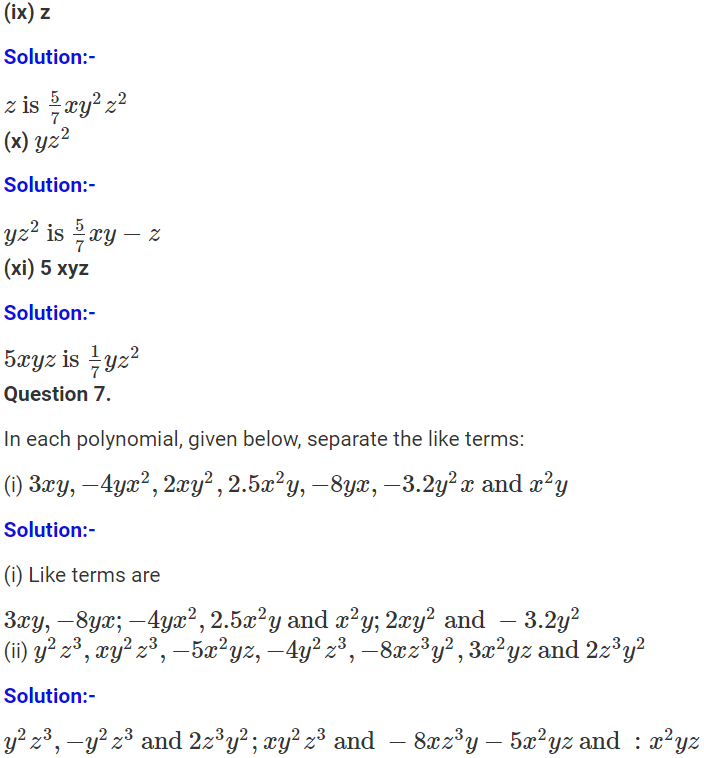Exercise 11B

Question 1

Evaluate: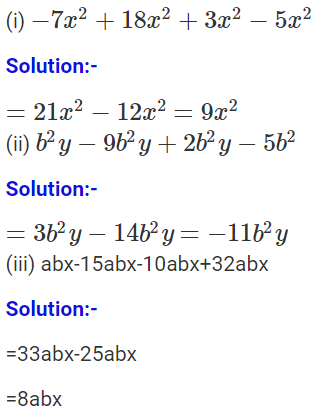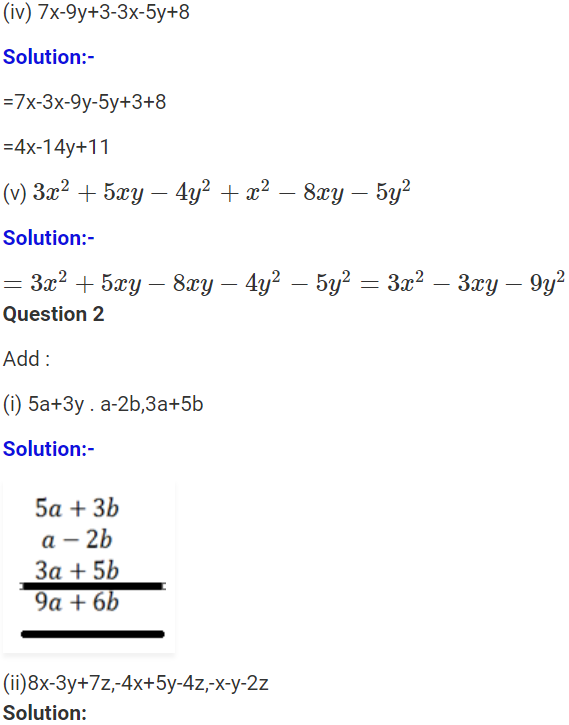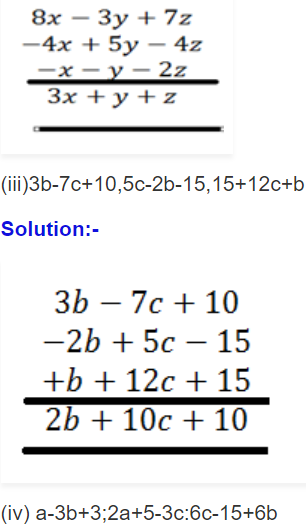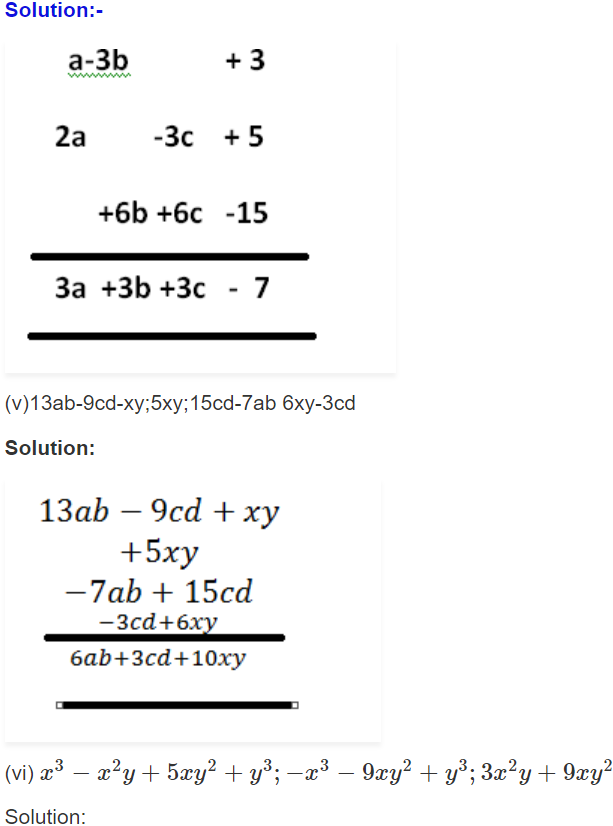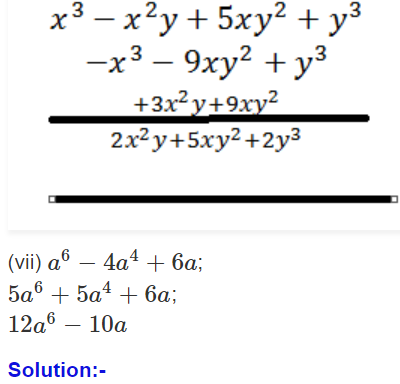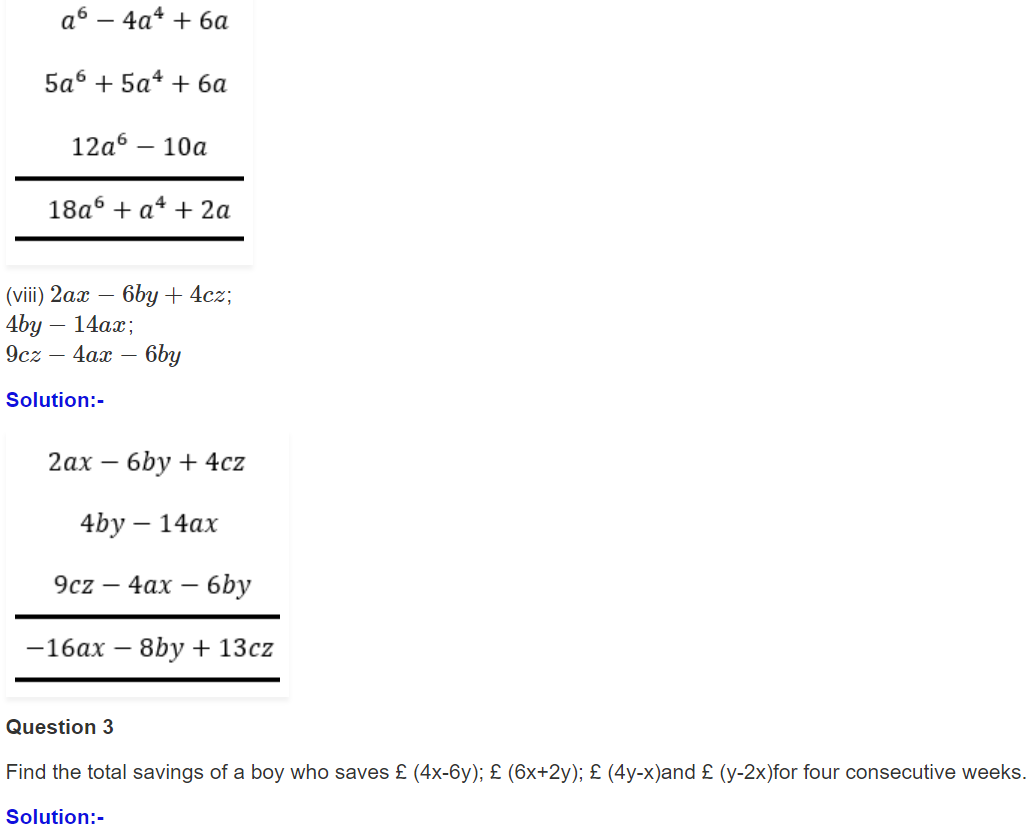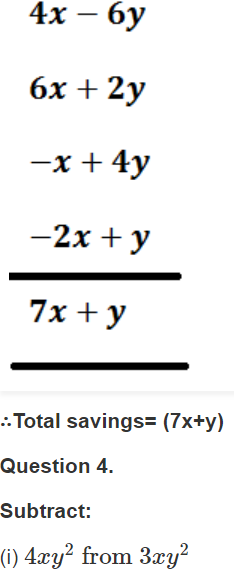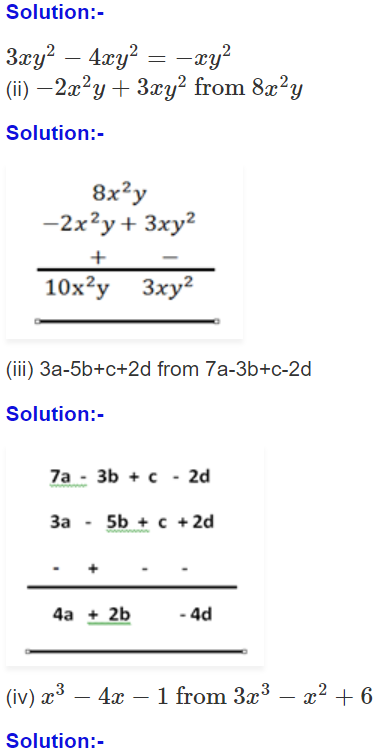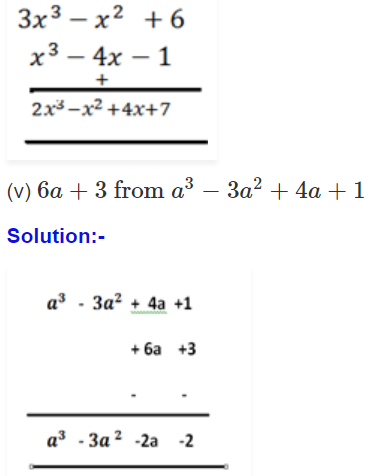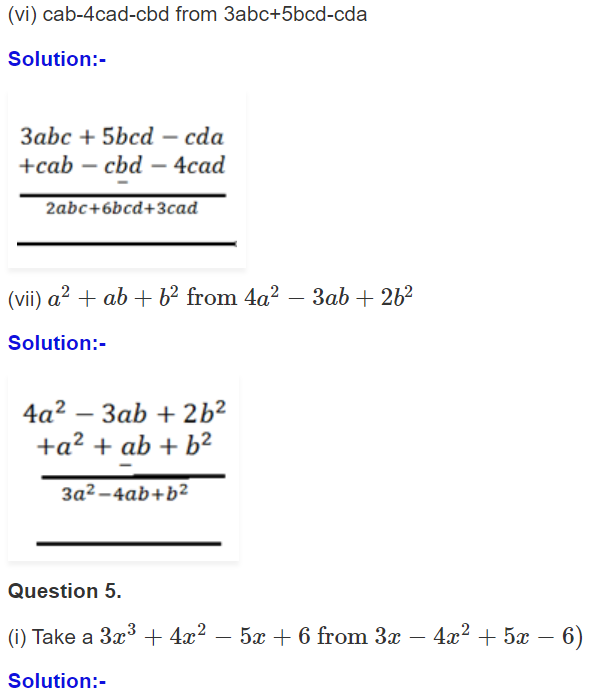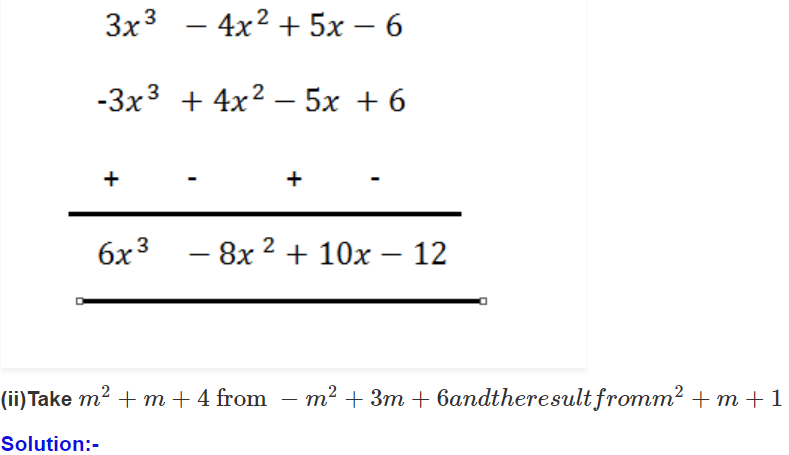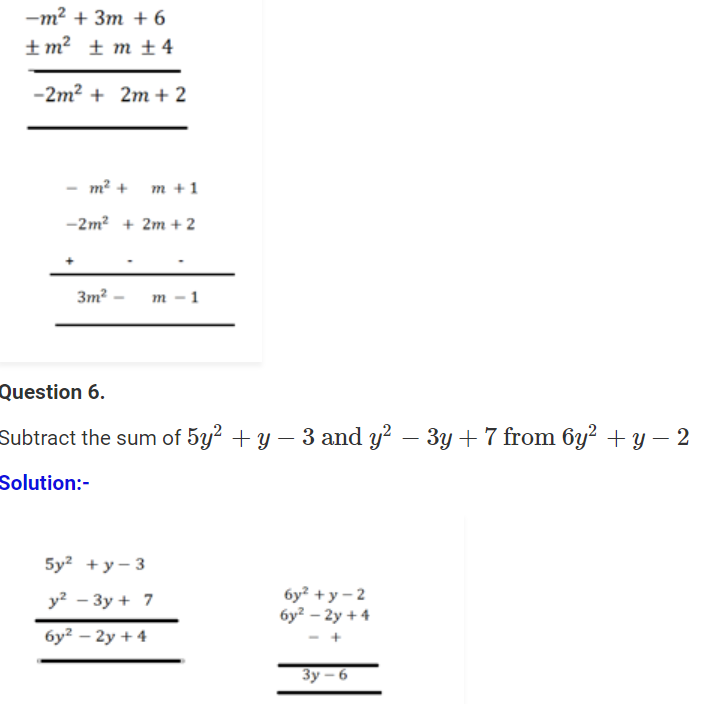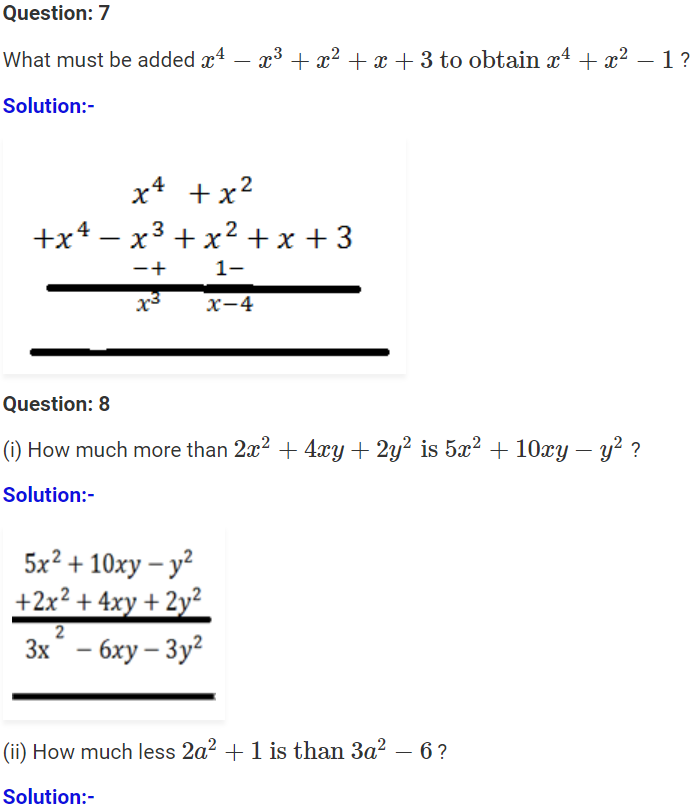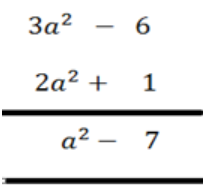Click on ICSE Class 8 Selina Solutions to get the answers for Maths, Physics, Chemistry and Biology subjects.

### Concepts Learned in ICSE Class 8 Maths Chapter 11 – Algebraic Expressions

In this chapter students have studied the following new terms and concepts:

1. Monomial: Expression that contains only one term is called a monomial.
2. Binomial: Expression that contains two terms is called a binomial.
3. Trinomial: An expression containing three terms is a trinomial.
4. Polynomial: an expression containing one or more terms with non-zero coefficients (with variables having non-negative exponents) is called a polynomial.
5. Definition of Degree and Coefficient along with the examples.
6. Addition and subtraction of algebraic expressions.

We hope this information on “ICSE Class 8 Maths Selina Solutions Chapter 11 Algebraic Expressions” is useful for students. Keep learning and stay tuned for further updates on ICSE and other competitive exams. To access interactive Maths and Science Videos download BYJU’S App and subscribe to YouTube Channel.